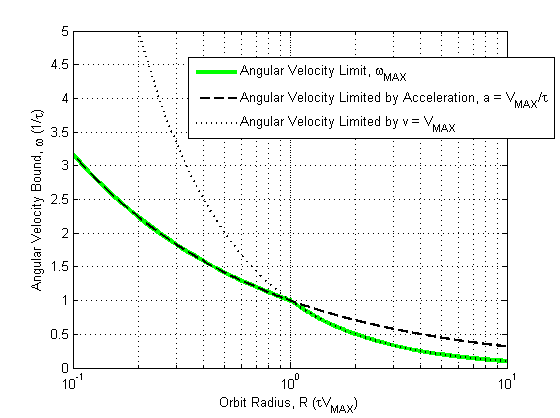## Thursday, October 29, 2015

### Refinement to Fundamental Limits of Orbits

My recent travels for EVE Vegas 2015 reminded me of the benefits to my concentration of doing math in the airport while waiting for a flight.  In the midst of sounds from airport slot machines and tourists from the mid-west comparing who hates Obama the most, I had a moment of clarity on the subject of asymptotic orbit performance.  This avenue of inquiry was covered in Part II, but I am revisiting it with the loose orbit case now that my new blog post on manual orbit is nearing completion.  So this post is just a minor refinement to the manual orbit section I presented in Part II.

In EVE's motion model, ships are limited in their maximum velocity.  They are also limited in the maximum acceleration they can achieve.  I made a point of emphasizing this in my notes in Part II.  When it came to bounding manual orbit performance, however, I bounded the maximum angular velocity on orbits that were limited by acceleration only, and I didn't consider that there is a point at which the velocity of the ship cannot increase past VMAX.

Just for review, recall that a stable orbit is characterized by a balance between centripetal force and the acceleration force that a ship can achieve.
$\large F_{centripetal} = F_{ship}$

I know that the maximum acceleration a ship can produce is VMAX/τ, so I can solve for the relationship between velocity and orbit distance,
$\large F_{centripetal} = \frac{M v^2}{R}$
$\large F_{ship,MAX} = M\frac{V_{MAX}}{\tau}$

Isolating angular velocity by writing the ratio v/R
$\large \omega_{accel,limit} = \frac{v}{R} = \sqrt{\frac{V_{MAX}}{\tau R}}$                               (10.26-1)

This is what I did in the chapter, however, notice that if I write just the velocity from (10.26-1), beyond a orbit radius of τVMAX, the velocity of the ship would need to be greater than VMAX!  This is obviously not possible, unless there are some external forces acting on the ship.

So, if we aren't limited by our ship's maximum acceleration when orbiting beyond the characteristic distance, then the obvious limit in this region is the ship's maximum velocity.  That is,
$\large \omega_{V_{MAX},limit} \leq \frac{V_{MAX}}{R}$                          (10.26-2)

This limit is obvious, but it is worth correcting since my original writing did not acknowledge this boundary.  The updated bound for angular velocity is now,
$\large \omega_{maximum} \leq \begin{cases} \sqrt{\frac{V_{MAX}}{\tau R}}, & \text{ if } R \leq \tau V_{MAX} \\ \frac{V_{MAX}}{R}, & \text{ if } R > \tau V_{MAX} \end{cases}$        (10.26-3)

Another minor note I want to point out is the boundary between these two conditions.  An orbit at the characteristic distance, τVMAX, has a limit on angular velocity of 1/τ.  This point is an easy one to remember, so I'm going to use it to distinguish between 'tight' orbits and 'loose' orbits.  An orbit with radius closer than the characteristic distance of your ship is limited by your acceleration towards the center of the orbit, which means that manual orbiting techniques are likely to be of value.

Below I present a normalized graph showing how the new bound for maximum velocity affects the limit on angular velocity proposed for any ship for parameters VMAX and τFigure 10.26-1:  I have graphed the limits to angular velocity normalized to ship motion parameters, and now based on two limitations of ship motion - maximum acceleration and maximum forward velocity.  This graph is normalized to distance units of τVMAX in orbit distance, and (1/τ) in angular velocity, so that at an orbit distance of τVMAX the rate is limited to (1/τ).

Now that I have cleared up the limitations to orbit performance with Equation (10.26-3), I can go back and plot the existing ship data against this limit.  The original figure shows poor agreement between measured orbit angular velocity when the orbit radius is greater than τVMAX.  In the figure below, I have updated the theoretical limit traces to include the velocity limitation.  You can see that in the AB and No Prop. Mod. cases, the angular velocity approaches the theoretical.  This is also true in the case when MWD propulsion mods are used because the angle to the acceleration point is almost the same as accelerating towards the center of the orbit circle.Figure 10.26-2: Enyo automatic orbit data for No Prop. Mod., AB, and MWD are compared with theoretical limits. Note that these points correspond to orbit commands for 500m and at intervals up to 7500m.

These tighter bounds on orbit performance will be helpful background when I present one possible approach to manual orbits soon (TM).

### Interlude

I will be including a musical interlude with each blog post as an instructional aid.  I hope that you will find that it brings much clarity to your thinking.  Use caution when listening at elevated volumes or in populated areas.